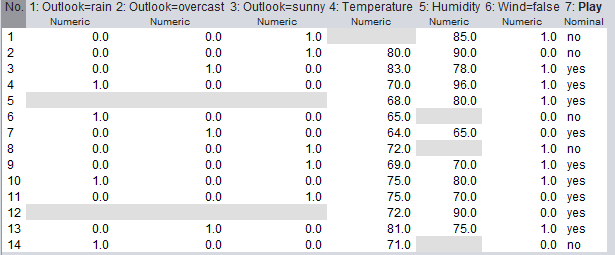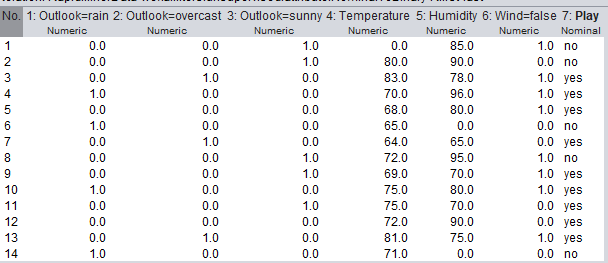# how does

Member Posts: 2Contributor I
edited June 2019 in Help
SOLVED
========
In WEKA, MLP classifier automatically ignore the missing value, but i don't know how it works.
I've read this http://weka.8497.n7.nabble.com/How-does-MultilayerPerceptron-classifier-handle-the-missing-value-tt44918.html , it says "missing values are assumed to be 0 in MultilayerPerceptron", so does that mean if there missing values, it will be replaced by 0 value ?
but when I replace missing values with 0 value, the result instead give reduced accuracy in WEKA.

here my practice:
I have dataset that preprocessed by NominalToBinary filter:then I use MLP classifier, the WEKA give me  64.2857 % accuracy:
```=== Stratified cross-validation ===
=== Summary ===

Correctly Classified Instances           9               64.2857 %
Incorrectly Classified Instances         5               35.7143 %
Kappa statistic                          0
Mean absolute error                      0.4762
Root mean squared error                  0.4934
Relative absolute error                100      %
Root relative squared error            100      %
Total Number of Instances               14

=== Detailed Accuracy By Class ===

TP Rate  FP Rate  Precision  Recall   F-Measure  MCC      ROC Area  PRC Area  Class
0.000    0.000    ?          0.000    ?          ?        0.178     0.318     no
1.000    1.000    0.643      1.000    0.783      ?        0.178     0.555     yes
Weighted Avg.    0.643    0.643    ?          0.643    ?          ?        0.178     0.470

=== Confusion Matrix ===

a b   <-- classified as
0 5 | a = no
0 9 | b = yes```
then I replaced missing values with 0 value:I do MLP classifier again, then WEKA give me 57.1429 % accuracy, lower accuracy than the dataset with missing value:
```=== Stratified cross-validation ===
=== Summary ===

Correctly Classified Instances           8               57.1429 %
Incorrectly Classified Instances         6               42.8571 %
Kappa statistic                          0.0667
Mean absolute error                      0.3973
Root mean squared error                  0.5731
Relative absolute error                 83.4356 %
Root relative squared error            116.169  %
Total Number of Instances               14

=== Detailed Accuracy By Class ===

TP Rate  FP Rate  Precision  Recall   F-Measure  MCC      ROC Area  PRC Area  Class
0.400    0.333    0.400      0.400    0.400      0.067    0.667     0.481     no
0.667    0.600    0.667      0.667    0.667      0.067    0.667     0.850     yes
Weighted Avg.    0.571    0.505    0.571      0.571    0.571      0.067    0.667     0.718

=== Confusion Matrix ===

a b   <-- classified as
2 3 | a = no
3 6 | b = yes```

so i don't think "Ignore missing value" same as replace the missing value with 0 value. So can you explain to me how "Ignore missing value" actually work in MLP classifier ? and how apply it in Neural Network operator in RapidMiner ?